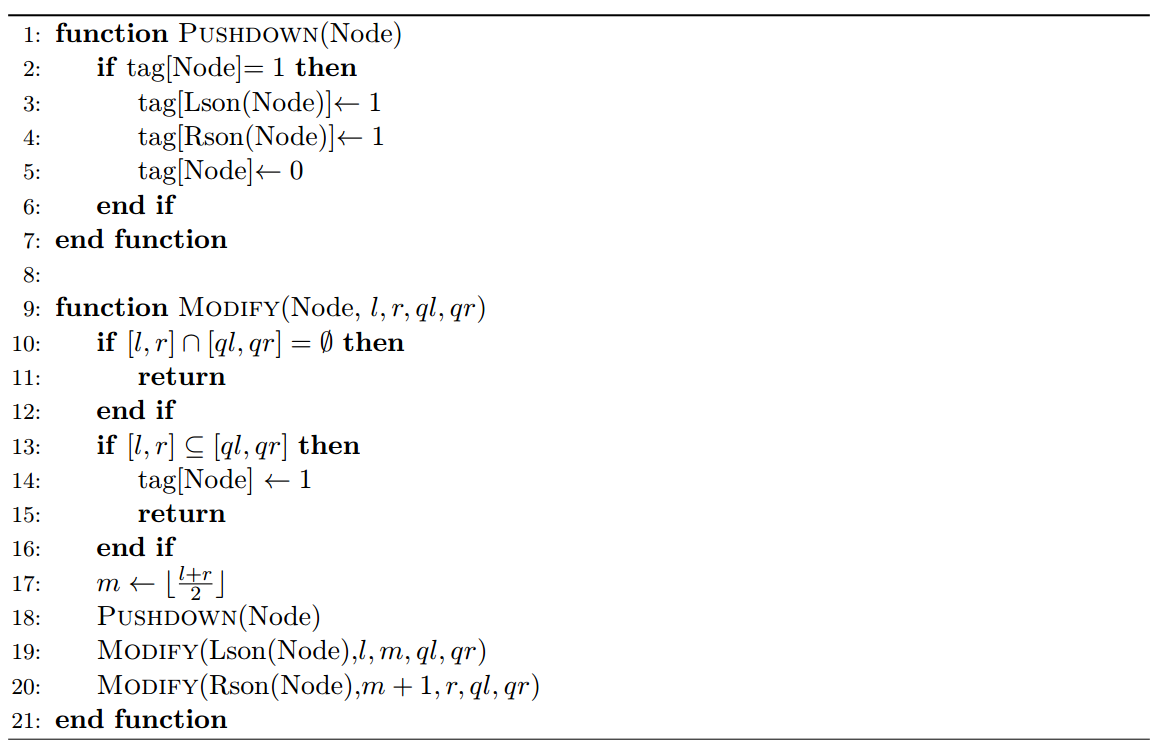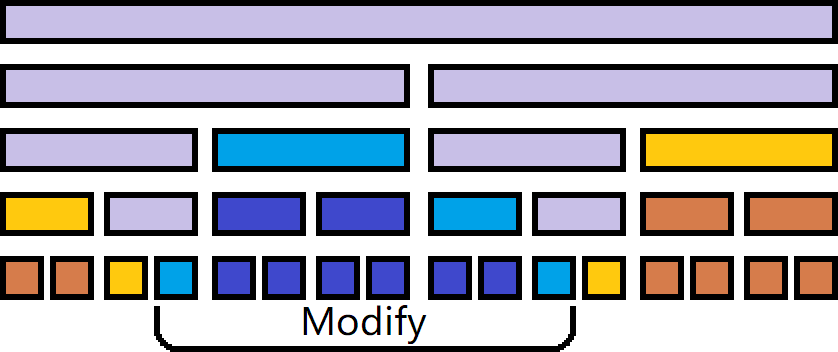# LOJ 3043: 洛谷 P5280: 「ZJOI2019」线段树

### 题意简述：### 题解：

1. 与修改区间相交，但不包含在修改区间内部的节点（浅紫色）。

2. 包含在修改区间内部，但其父亲不存在或不包含在修改区间内部（浅蓝色）。

3. 与修改区间相离，但其父亲与修改区间相交（浅橙色）。

4. 包含在修改区间内部，且其父亲也包含在修改区间内部（深蓝色）。

5. 与修改区间相离，且其父亲也与修改区间相离（深橙色）。$5$ 类节点的信息没有更改，第 $4$ 类节点仅有 $\mathrm{g}$ 有更改，因为第 $4$ 类节点有 $\mathcal{O}(n)$ 个，所以必须采用打懒标记的方法来维护。

#include <cstdio>

typedef long long LL;
const int Mod = 998244353;
const int Inv2 = 499122177;
const int MS = 1 << 18;

inline int Add(int x, int y) {
return (x += y) >= Mod ? x - Mod : x;
}

int N, M;

int f[MS], g[MS], Sf[MS], T[MS];
inline void P(int i, int x) {
g[i] = ((LL)g[i] * x + 1 - x + Mod) % Mod;
T[i] = (LL)T[i] * x % Mod;
}
inline void Upd(int i, int Ty) {
if (Ty) Sf[i] = f[i];
else Sf[i] = Add(f[i], Add(Sf[i << 1], Sf[i << 1 | 1]));
}
inline void Psd(int i) {
P(i << 1, T[i]);
P(i << 1 | 1, T[i]);
T[i] = 1;
}
void Build(int i, int l, int r) {
T[i] = 1;
if (l != r) {
Build(i << 1, l, (l + r) >> 1);
Build(i << 1 | 1, ((l + r) >> 1) + 1, r);
}
}
void Mdf(int i, int l, int r, int a, int b) {
if (r < a || b < l) {
f[i] = (LL)(f[i] + g[i]) * Inv2 % Mod;
Upd(i, l == r);
return ;
}
if (a <= l && r <= b) {
f[i] = (LL)(f[i] + 1) * Inv2 % Mod;
Upd(i, l == r);
P(i, Inv2);
return ;
}
Psd(i);
f[i] = (LL)f[i] * Inv2 % Mod;
g[i] = (LL)g[i] * Inv2 % Mod;
Mdf(i << 1, l, (l + r) >> 1, a, b);
Mdf(i << 1 | 1, ((l + r) >> 1) + 1, r, a, b);
Upd(i, 0);
}

int main() {
scanf("%d%d", &N, &M);
Build(1, 1, N);
for (int m = 1, C = 1; m <= M; ++m) {
int op, l, r;
scanf("%d", &op);
if (op == 1) {
scanf("%d%d", &l, &r);
Mdf(1, 1, N, l, r);